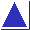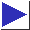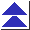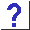# comp.lang.c FAQ list ·Question 15.11

Q: I can't get va_arg to pull in an argument of type pointer-to-function.

A: Try using a typedef for the function pointer type.

The type-rewriting games which the va_arg macro typically plays are stymied by overly-complicated types such as pointer-to-function. To illustrate, a simplified implementation of va_arg is

```	#define va_arg(argp, type) \
(*(type *)(((argp) += sizeof(type)) - sizeof(type)))
```
where argp's type (va_list) is char *. When you attempt to invoke
```	va_arg(argp, int (*)())
```
the expansion is
```	(*(int (*)() *)(((argp) += sizeof(int (*)())) - sizeof(int (*)())))
```
which is a syntax error (the first cast (int (*)() *) is meaningless). [footnote]

If you use a typedef for the function pointer type, however, all will be well. Given

```	typedef int (*funcptr)();
```
the expansion of
```	va_arg(argp, funcptr)
```
is
```	(*(funcptr *)(((argp) += sizeof(funcptr)) - sizeof(funcptr)))
```
which works correctly.